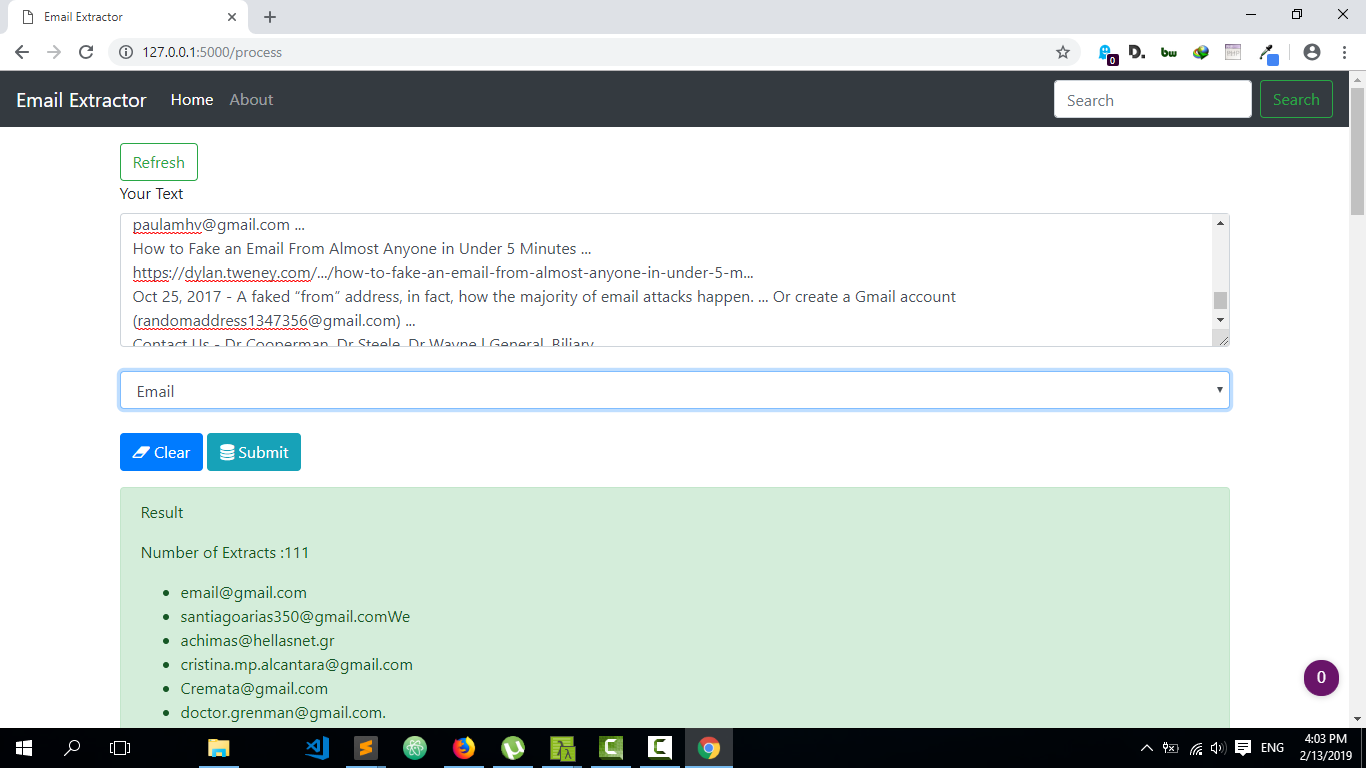# Building An Email Extractor Web Application

### Building an Email ,Phone Number and URL extractor web application

In this tutorial we will build a simple web application for extracting email,phone numbers and urls in a simplified way. We will be using flask as our back-end and bootstrap as our front-end frameworks.The main idea is to use regular expression to extract our email,phone number and url links. Below is the required regular expressions for doing the extraction.Note that these are simple regex, you can add complex regex for more efficient extraction.

Email: r”[\w\.-]+@[\w\.-]+”

Phone number: r’\d\d\d.\d\d\d.\d\d\d\d’

URL/HTTP: rhttp?://www\.?\w+\.\w+

URL/HTTPS: rhttps?://www\.?\w+\.\w+

We will be using flask for our back-end.Below is the code

``````
# Regex
import re

email_regex = re.compile(r"[\w\.-]+@[\w\.-]+")
phone_num = re.compile(r'\d\d\d.\d\d\d.\d\d\d\d')
url_https_regex = re.compile(r"https?://www\.?\w+\.\w+")
url_regex = re.compile(r"http?://www\.?\w+\.\w+")

# Initialize app

# Route
@app.route('/')
def index():
return render_template("index.html")

@app.route('/process',methods=['GET','POST'])
def process():
if request.method == 'POST':
if choice == 'email':
rawtext = request.form['rawtext']
results = email_regex.findall(rawtext)
num_of_results = len(results)
elif choice == 'phone':
rawtext = request.form['rawtext']
results = phone_num.findall(rawtext)
num_of_results = len(results)
elif choice == 'url_https':
rawtext = request.form['rawtext']
results = url_https_regex.findall(rawtext)
num_of_results = len(results)
elif choice == 'url':
rawtext = request.form['rawtext']
results = url_regex.findall(rawtext)
num_of_results = len(results)

return render_template("index.html",results=results,num_of_results=num_of_results)

if __name__ == '__main__':
app.run(debug=True)

``````

To be able to get input from our front-end to our back-end we will be using a basic form input and send the data via post to our flask route “process” where the data would be processed and return to our front end html page.

You can get the complete code here

Below is a video tutorial demonstrating the entire process.